# Logical AND, OR, NOT Operators in Oracle

## Logical AND OR & NOT Operators in Oracle with Examples

In this article, I am going to discuss Logical Operators (AND OR & NOT) in Oracle with Examples. Please read our previous article, where we discussed Relational Operators in Oracle with Examples.

##### What are Logical Operators in Oracle?

If you want to combine more than one condition, then you need to use the Logical Operators in Oracle. The Logical Operators in Oracle are basically used to check for the truth-ness of some conditions. Logical operators return a Boolean data type with a value of TRUE, or FALSE. In Oracle, there are three Logical Operators available. They are as follows:

1. AND: TRUE if both Boolean expressions are TRUE.
2. OR: TRUE if one of the Boolean expressions is TRUE.
3. NOT: Reverses the value of any other Boolean operator.

The Logical Operators in Oracle are used to compare two conditions to check whether a row (or rows) can be selected for the output.

##### Logical Operators Examples in Oracle:

Let us understand how to use Logical Operators in Oracle with Examples. We are going to use the following Employee table to understand the Logical Operators.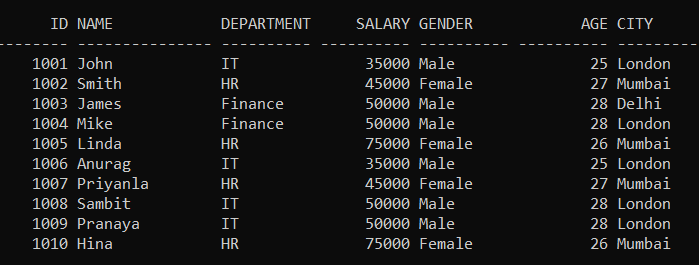Please execute the below SQL query to drop the existing Employee table and Create a new Employee table with the required sample data. We also set the linesize to get the output in the above format.

```SET linesize 300;

DROP Table Employee;

CREATE TABLE Employee (
Id INT PRIMARY KEY,
Name VARCHAR(15),
Department VARCHAR(10),
Salary NUMBER(8, 2),
Gender VARCHAR(10),
Age INT,
City VARCHAR(10)
);

INSERT INTO Employee (Id, Name, Department, Salary, Gender, Age, City) VALUES (1001, 'John', 'IT', 35000, 'Male', 25, 'London');
INSERT INTO Employee (Id, Name, Department, Salary, Gender, Age, City) VALUES (1002, 'Smith', 'HR', 45000, 'Female', 27, 'Mumbai');
INSERT INTO Employee (Id, Name, Department, Salary, Gender, Age, City) VALUES (1003, 'James', 'Finance', 50000, 'Male', 28, 'Delhi');
INSERT INTO Employee (Id, Name, Department, Salary, Gender, Age, City) VALUES (1004, 'Mike', 'Finance', 50000, 'Male', 28, 'London');
INSERT INTO Employee (Id, Name, Department, Salary, Gender, Age, City) VALUES (1005, 'Linda', 'HR', 75000, 'Female', 26, 'Mumbai');
INSERT INTO Employee (Id, Name, Department, Salary, Gender, Age, City) VALUES (1006, 'Anurag', 'IT', 35000, 'Male', 25, 'London');
INSERT INTO Employee (Id, Name, Department, Salary, Gender, Age, City) VALUES (1007, 'Priyanla', 'HR', 45000, 'Female', 27, 'Mumbai');
INSERT INTO Employee (Id, Name, Department, Salary, Gender, Age, City) VALUES (1008, 'Sambit', 'IT', 50000, 'Male', 28, 'London');
INSERT INTO Employee (Id, Name, Department, Salary, Gender, Age, City) VALUES (1009, 'Pranaya', 'IT', 50000, 'Male', 28, 'London');
INSERT INTO Employee (Id, Name, Department, Salary, Gender, Age, City) VALUES (1010, 'Hina', 'HR', 75000, 'Female', 26, 'Mumbai');

```
##### Logical AND Operator in Oracle

The Logical AND operator in Oracle compares two conditions and returns TRUE if both of the conditions are TRUE and returns FALSE when either is FALSE. If you want to select rows that must satisfy all the given conditions, then in such cases you need to use the AND operator in Oracle.

In some cases, there are chances where we will have to use more than one condition to filter the data rows. In such a case, we use some special operators that are useful to create such compound conditions. The AND operator is useful to add multiple conditions in a single SQL statement. It only displays the data rows if all conditions are TRUE. If any one of the conditions is false the SQL statement will return an empty result set.

We can use AND condition with SELECT, INSERT, UPDATE or DELETE statements to test two or more conditions in an individual query.

##### Syntax to use AND Operator in Oracle: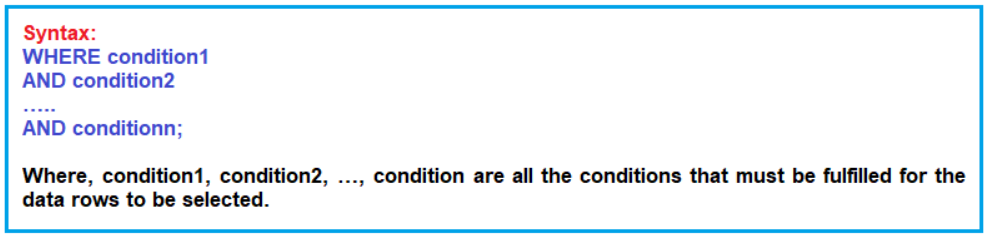Note: Returns ‘True’ if both component conditions are true. Returns ‘False’ if any one component condition or Both Component conditions are False.

##### Examples to Understand Logical AND Operator in Oracle:

Let’s see some examples to understand the need and use of AND operator in Oracle. Adding the conditions in the bracket is optional. Using brackets for the conditions makes the code neat and clean.

Our requirement is to fetch all employees whose department is IT and Gender is Male. Now we want to filter the data rows with two conditions simultaneously using AND operator. Our requirement is to find all the employees from the Employee table where the Department is IT and the employee Gender is Male, then we need to use the AND operator as shown in the below query.

```SELECT * FROM employee WHERE (department = 'IT' AND Gender = 'Male'); --OR
SELECT * FROM employee WHERE department = 'IT' AND Gender = 'Male'; -- Bracket is optional```

When you run the above SELECT statement, the system evaluates if the first condition, that is department value equal to IT is true. If the first condition is true the system, then evaluates the second condition i.e. Gender is Male. If both first and second conditions are true the system returns the data row. If any one of the conditions is false the system won’t return that data row. As in our Employee table, four employees satisfy the above two conditions, so when you execute the above query, you will get the following data rows as the output.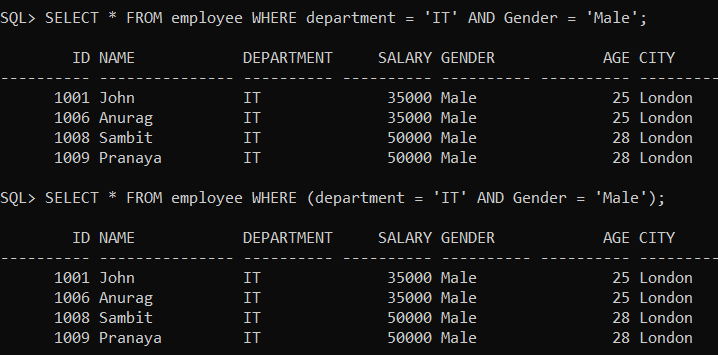##### False Condition Example using AND Operator in Oracle:

Let’s modify the SQL statement so that the second condition becomes false. As in our employee table, there is no employee in the IT department whose age is 28. Now, in the below SQL Statement, the second condition becomes false.

SELECT * FROM Employee WHERE Department = ‘IT’ AND Age = 26;

When you execute the above SQL query, then you will not get any data rows as shown in the below image. And this time you can see, even though we have data rows with department values equal to IT, we got an empty result set, as the second condition age=26 was never true for any row.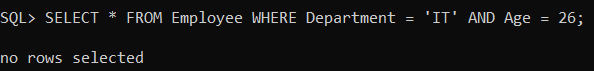##### Logical NOT Operator Example in Oracle:

We can also use the NOT keyword in the statement to revert one of the conditions. Suppose our requirement is to fetch all the employees whose Department is IT and Age is not equal to 28. Then in that case we can use NOT Operator as shown in the below query.

SELECT * FROM employee WHERE department = ‘IT’ AND NOT Age = 28;

When you execute the above SQL query, you will get the following output. And this time, the result set contains only the data rows where the age column value is not equal to 28 and the department column value is IT as shown in the below image.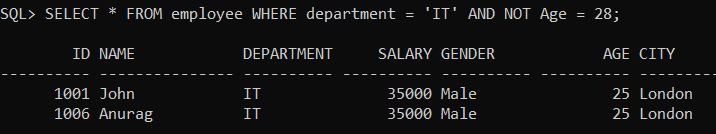##### Logical OR Operator in Oracle

In some cases, we will have to evaluate only one of the conditions is TRUE to return the result set. In such a case, the logical OR operator is useful to create such compound conditions. Similar to AND operator, The OR operator in Oracle is useful to add multiple conditions in a single SQL statement. It displays the data rows if any one of the multiple conditions is TRUE. If all the conditions are false the SQL statement won’t return any result set.

Adding the conditions in the bracket is optional. Using brackets for the conditions makes the code neat and clean. The following is the syntax to use the logical OR operator in MySQL.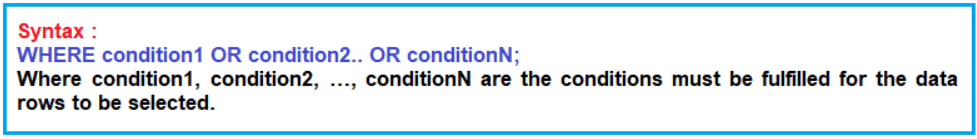Note: Returns True if either component conditions become TRUE. Returns False if both the component conditions become False.

##### Example: Fetch all the Employees whose age is either 25 or 26 from the Employee table.

Now we will filter the data rows with two conditions simultaneously using the “OR” operator. Our requirement is to fetch all the employees from the Employee table whose age is either 25 or 26, then we need to use the OR operator as shown in the below query.

```SELECT * FROM Employee WHERE age = 25 OR age = 26; -- OR
SELECT * FROM Employee WHERE (age = 25 OR age = 26); -- Bracket is optional```

When we run the above SQL statement the system evaluates if the first condition, that is age = 25 is true. Whether the condition is true or false, the system evaluates the second condition. If any of the conditions is true the system returns the data row. If all the conditions are false the system won’t return that data row. Now run the statement, and you can see, the system returned only the data rows where the age value is 25 or 26 as shown in the below image.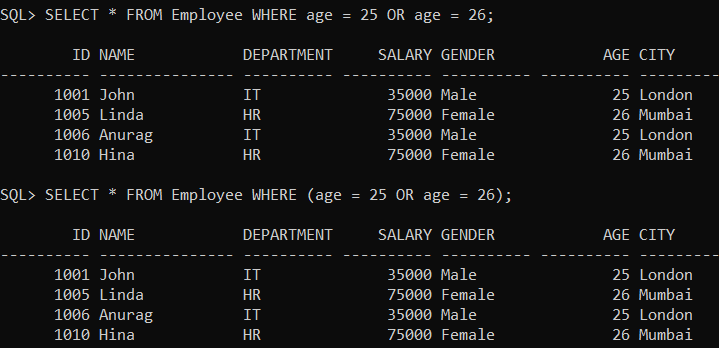Let’s modify the SQL statement so that both conditions become FALSE. We will change the first condition to age = 10 and the second condition to age = 15. There is no record with the “age” column with a value of 10 or 15, so both the conditions will be false.

SELECT * FROM Employee WHERE Age = 10 OR Age = 15;

Run the above SQL statement. And this time you will get an empty result set as shown in the below image.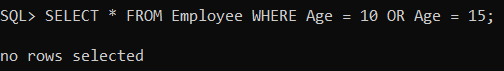##### Logical NOT Operator in Oracle:

The Logical NOT Operator in Oracle takes a single Boolean as an argument and changes its value from false to true or from true to false. If we want to select rows that do not satisfy a condition, then you need to use the logical NOT operator. NOT results in the reverse of a condition. That is, if a condition is satisfied, then the row is not returned. For example, if we want to fetch the Employees who do not belong to the City of London, then we need to use the NOT Operator as shown in the below SQL query.

SELECT * FROM Employee WHERE NOT City = ‘London’;

When you execute the above SQL Query, it will give you the below result set. As you can see, the result set excludes the London city employees.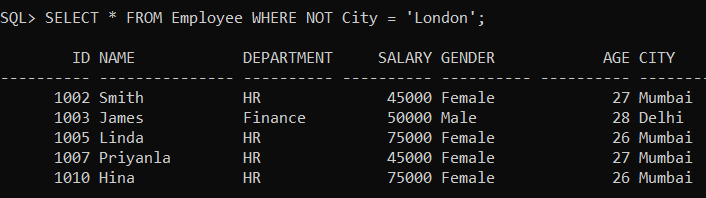Note: The NOT operator returns True if the condition is False and returns ‘False’ if the following condition is True.

##### Nested Logical Operators in Oracle:

We can also use multiple logical operators in a single SQL statement in Oracle. When we combine the logical operators in a SELECT statement, the order in which the statement is processed is

1. NOT
2. AND
3. OR
##### Nested Logical Operators Example:

If we want to select the employees whose Salary is between 27000 and 30000, or those whose City is not Mumbai, then the query would be like,

SELECT * FROM Employee WHERE Salary >= 27000 AND Salary <= 30000 OR NOT CITY = ‘Mumbai’;

When you execute the above SQL Query, it will give you the below result set.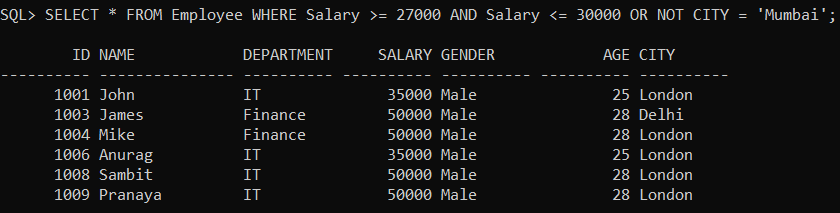In this case, the filter works as follows:

1. First, all the Employees who do not belong to the City of Mumbai are selected.
2. Second, all the Employees, whose Salary between 27000 and 30000 are selected.
3. And finally, the result is the rows that satisfy at least one of the above conditions are returned.

Note: The order of the condition is important, if the order changes we may get a different result.

In the next article, I am going to discuss IN Operator in Oracle with Examples. Here, in this article, I try to explain Logical Operator in Oracle with Examples and I hope you enjoy this Logical Operator in Oracle with Examples article.